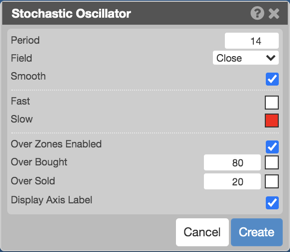Charts

# Stochastic Oscillator (STOCH)

The Stochastic Oscillator (Stoch) normalizes price as a percentage between 0 and 100. Normally two lines are plotted, the %K line and a moving average of the %K which is called %D. A slow stochastic can be created by initially smoothing the %K line with a moving average before it is displayed. The length of this smoothing is set in the Slow K Period. Without the initial smoothing ( i.e., setting the Slow K Period to a value of 1 ) the %K becomes the 'Raw %K' value, and is also known as a fast stochastic.## Configuration Options• Period: Number of bars to use in the calculations.
• Field: Price or combination of prices to use as the base for average calculations. Possible values include:
• Open
• High
• Low
• Close
• HL/2 $$\left ( \frac{High + Low}{2} \right )$$
• HLC/3 $$\left ( \frac{High + Low + Close}{3} \right )$$
• HLCC/4 $$\left ( \frac{High + Low + Close + Close}{4} \right )$$
• OHLC/4 $$\left ( \frac{Open + High + Low + Close}{4} \right )$$
• Smooth: Whether to smooth the curve.
• Color Selectors: Colors to use for graph elements.
• Over Zones Enabled: Whether to shade the area between the plot and the horizontal overbought and oversold levels.
• Over Bought: Overbought quantity
• Over Sold: Oversold quantity
• Display Axis Label: Whether to display the most recent value on the Y axis.

## Formula

Fast %K = 100 SMA ( ( ( Close - Low ) / ( High - Low ) ),Time Period )

Slow %K = SMA ( Fast %K, Kma )

Slow %D = SMA ( Slow %K, Dma )

Where:

• Close = the current closing price
• Low = the lowest low in the past n periods
• High = the highest high in the past n periods
• Kma = Period of Moving Average used to smooth the Fast %K Values
• Dma = Period of Moving Average used to smooth the Slow %K Values